# Python Program to Illustrate Different Set Operations

In this tutorial you will learn about the Python Program to Illustrate Different Set Operations and its application with practical example.

In this tutorial, we will learn to create a Python Program to Illustrate Different Set Operations using python Programming.

## Prerequisites

Before starting with this tutorial we assume that you are best aware of the following Python programming topics:

• Python Operator.
• Basic Input and Output
• Basic Python  programming.
• Python Data type.
• Python in-built Functions.
• Loops in Python.
• List in python.

## Different set operations

Following are  Different set of operation are.

 Intersection A∩B all elements which are in both A and B. Union A∪B all elements which are in either A or B (or both) Difference A−B all elements which are in A but not in B Complement A¯or AC all elements which are not in A

## Python Program to Illustrate Different Set Operations

In this program we will create a Python program to ‘Illustrate Different Set Operations’ . We would first declared and initialized the required variables. Next, we would prompt user to input the values  later we will find  Different Set Operations.

## Output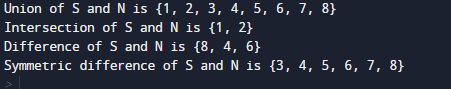In our program we will  ‘Illustrate Different Set Operations’ . We would first declared and initialized the required variables.First  we declared and initialized a set variables required in the program.

• S  and N= are two set elements.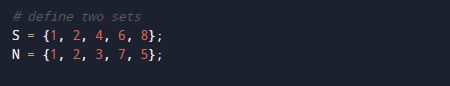We will demonstrates different operations set
Union of S and N is {1, 2, 3, 4, 5, 6, 7, 8}.
Intersection of S and N is {1, 2}.
Difference of S and N is {8, 4, 6}
Symmetric difference of S and N is {3, 4, 5, 6, 7, 8}.

Python Provide’s a Datatype called “Set” Elements within the “set” must be unique.So It can be used to perform different Operations like union, intersection, difference and symmetric difference.

Union.Intersection.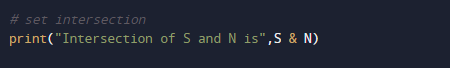Difference.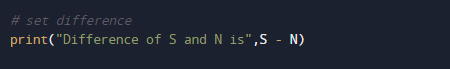Symmetric Difference.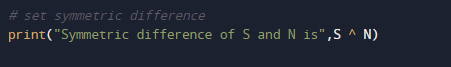In this program above, we have taken two different sets to perform different “set” operations on them.

In this tutorial we have learn about the Python Program to Illustrate Different Set Operations and its application with practical example. I hope you will like this tutorial.